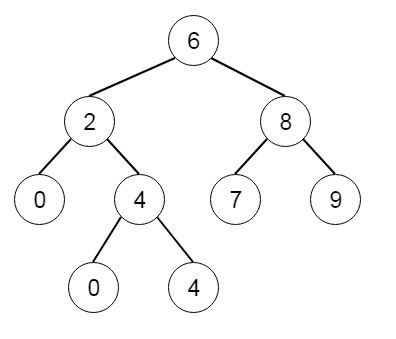# Lowest Common Ancestor of a Binary Search Tree in Python

Suppose we have a binary search tree. we have to find the Lowest common ancestor nodes of two given nodes. The LCA of two nodes p and q is actually as the lowest node in tree that has both p and q as decedent. So if the binary tree is like [6, 2, 8, 0, 4, 7, 9, null, null, 3, 5]. The tree will be like −Here LCA of 2 and 8 is 6

To solve this, we will follow these steps −

• If the tree is empty, then return null
• if p and q both are same as root, then return root
• left := LCA of left subtree of the root using p and q
• right := LCA of right subtree of the root using p and q
• if left and right both are non-zero, then return root
• return left OR right

## Example

Let us see the following implementation to get better understanding −

Live Demo

class TreeNode:
def __init__(self, data, left = None, right = None):
self.data = data
self.left = left
self.right = right
class Solution():
def lowestCommonAncestor(self, root, p, q):
if not root:
return None
if p == root or q==root:
return root
left = self.lowestCommonAncestor(root.left, p, q)
right = self.lowestCommonAncestor(root.right, p, q)
if left and right:
return root
return left or right
def insert(temp,data):
que = []
que.append(temp)
while (len(que)):
temp = que
que.pop(0)
if (not temp.left):
temp.left = TreeNode(data)
break
else:
que.append(temp.left)
if (not temp.right):
temp.right = TreeNode(data)
break
else:
que.append(temp.right)
def make_tree(elements):
Tree = TreeNode(elements)
for element in elements[1:]:
insert(Tree, element)
return Tree
def search_node(root, element):
if (root == None):
return None
if (root.data == element):
return root
res1 = search_node(root.left, element)
if res1:
return res1
res2 = search_node(root.right, element)
return res2

root = make_tree([6,2,8,0,4,7,9,None,None,3,5])
ob1 = Solution()
op = ob1.lowestCommonAncestor(root, search_node(root, 2), search_node(root, 8))
print(op.data)

## Input

[6,2,8,0,4,7,9,null,null,3,5]
2
8

## Output

6